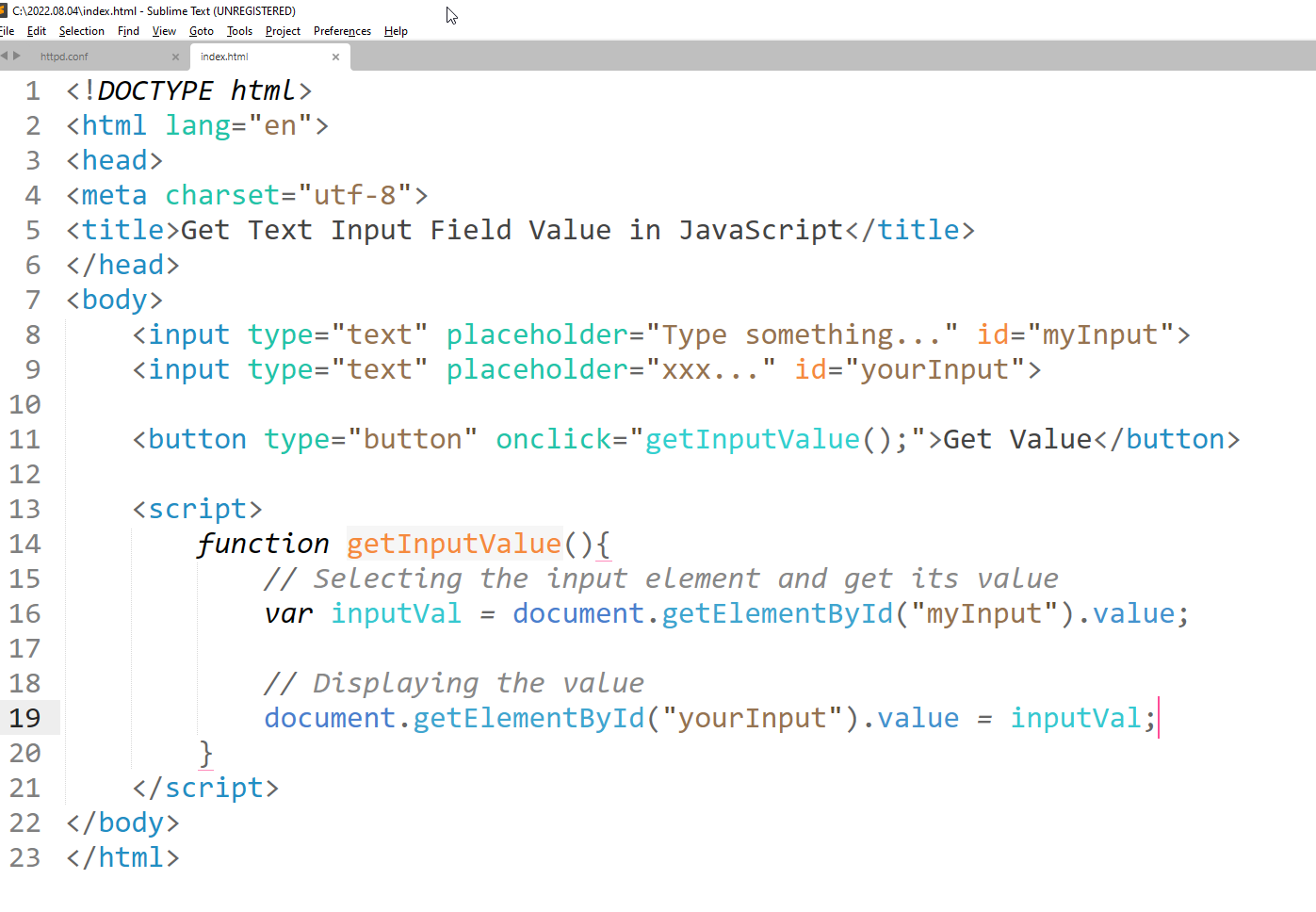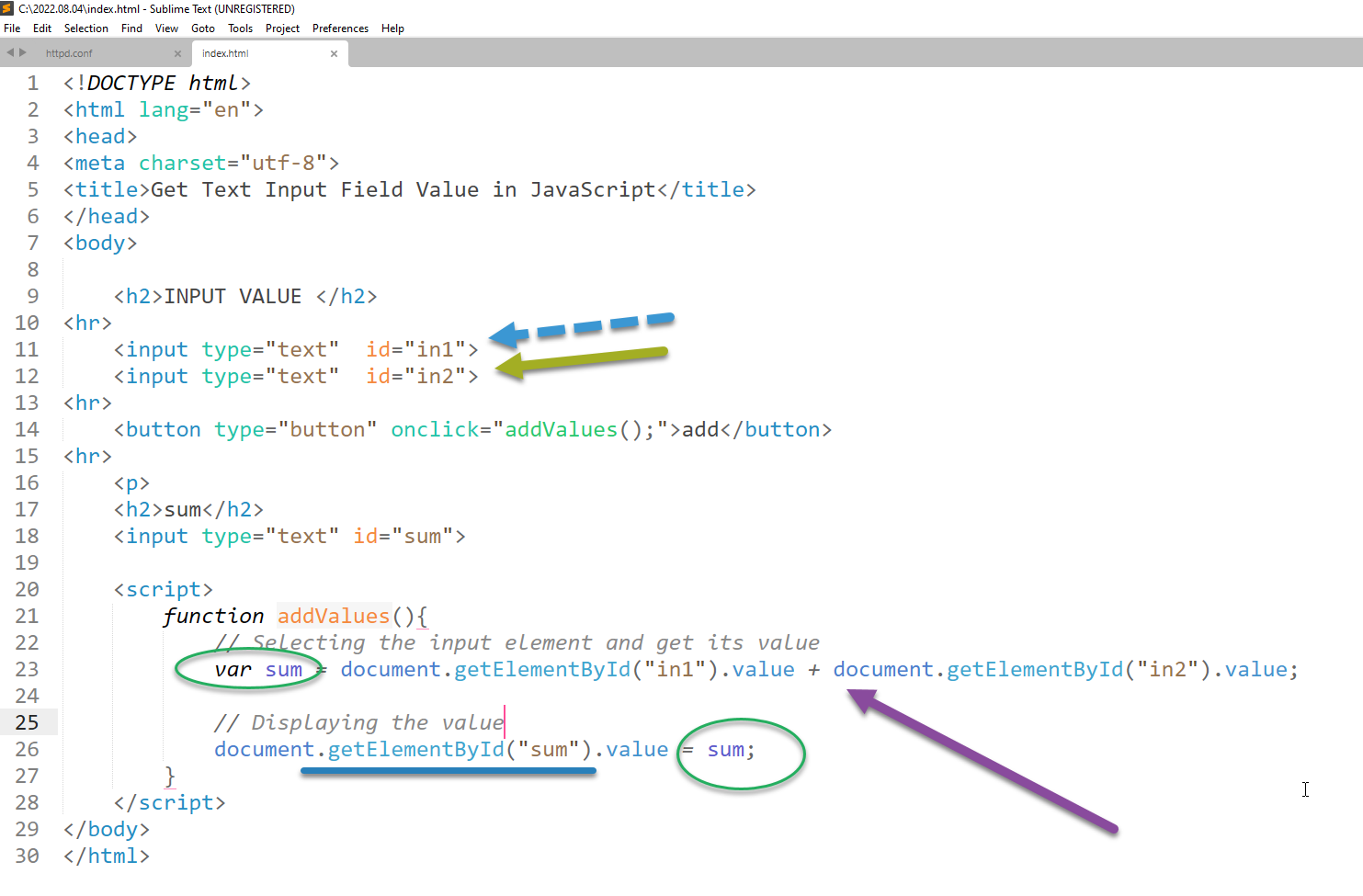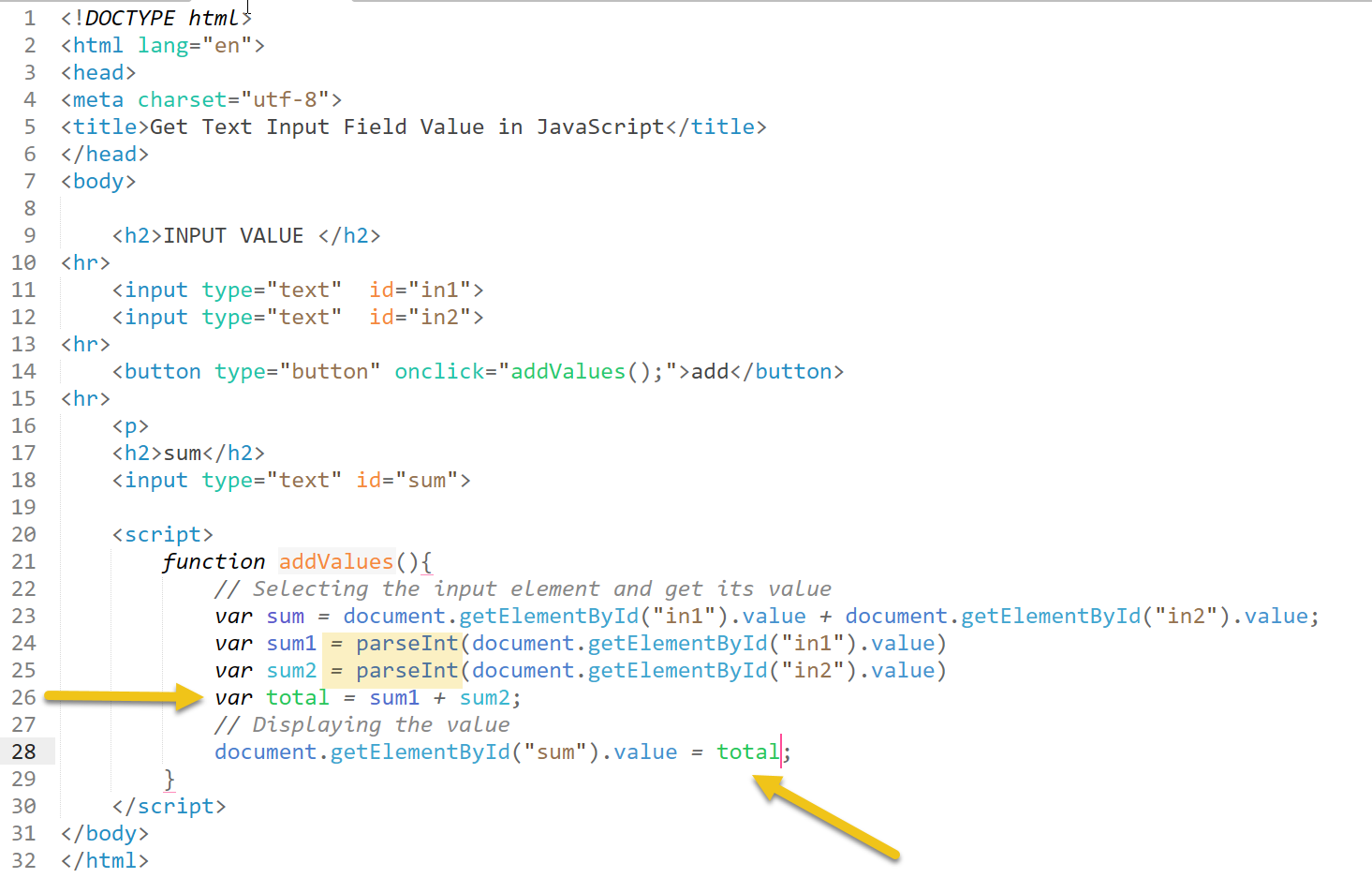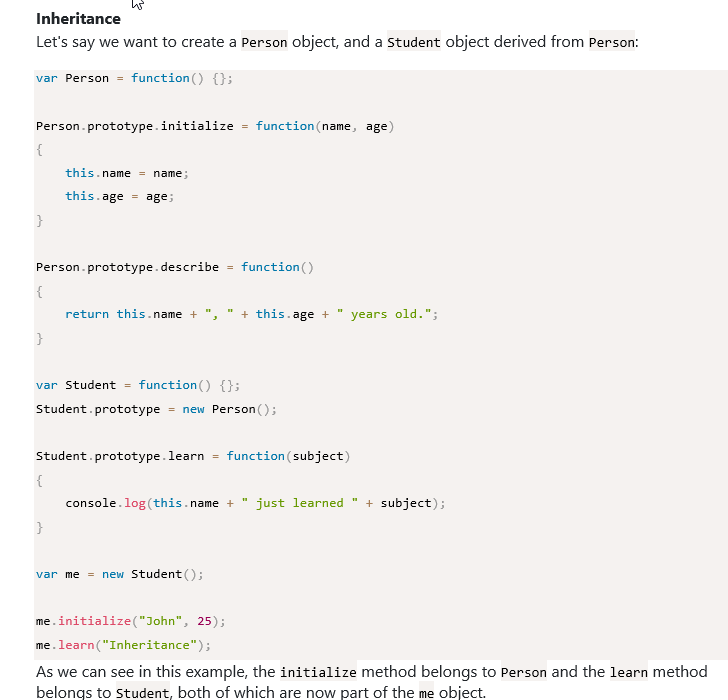Share
ExploreEnter this code and get it to run:A first solution to this probelm:## parseInt()#⁠⁠

The parseInt() method converts a string into an integer (a whole number).
It accepts two arguments. The first argument is the string to convert. The second argument is called the radix. This is the base number used in mathematical systems. For our use, it should always be 10.
`var text = '42px';``var integer = parseInt(text, 10);``// returns 42`

## parseFloat()#⁠⁠

The parseFloat() method converts a string into a point number (a number with decimal points). You can even pass in strings with random text in them.
`var text = '3.14someRandomStuff';``var pointNum = parseFloat(text);``// returns 3.14`

## Number()#⁠⁠

The Number() method converts a string to a number.
Sometimes it’s an integer. Other times it’s a point number. And if you pass in a string with random text in it, you’ll get NaN, an acronym for “Not a Number.”
As a result of this inconsistency, it’s a less safe choice than parseInt() and parseFloat(). If you know the format of the number you’d like, use those instead. If you want the string to fail with NaN if it has other characters in it, Number() may actually be a better choice.
`// Convert strings``Number('123'); // returns 123``Number('12.3'); // returns 12.3``Number('3.14someRandomStuff'); // returns NaN``Number('42px'); // returns NaN`
`⁠⁠⁠`
Implementing Inheritance in JavaScript